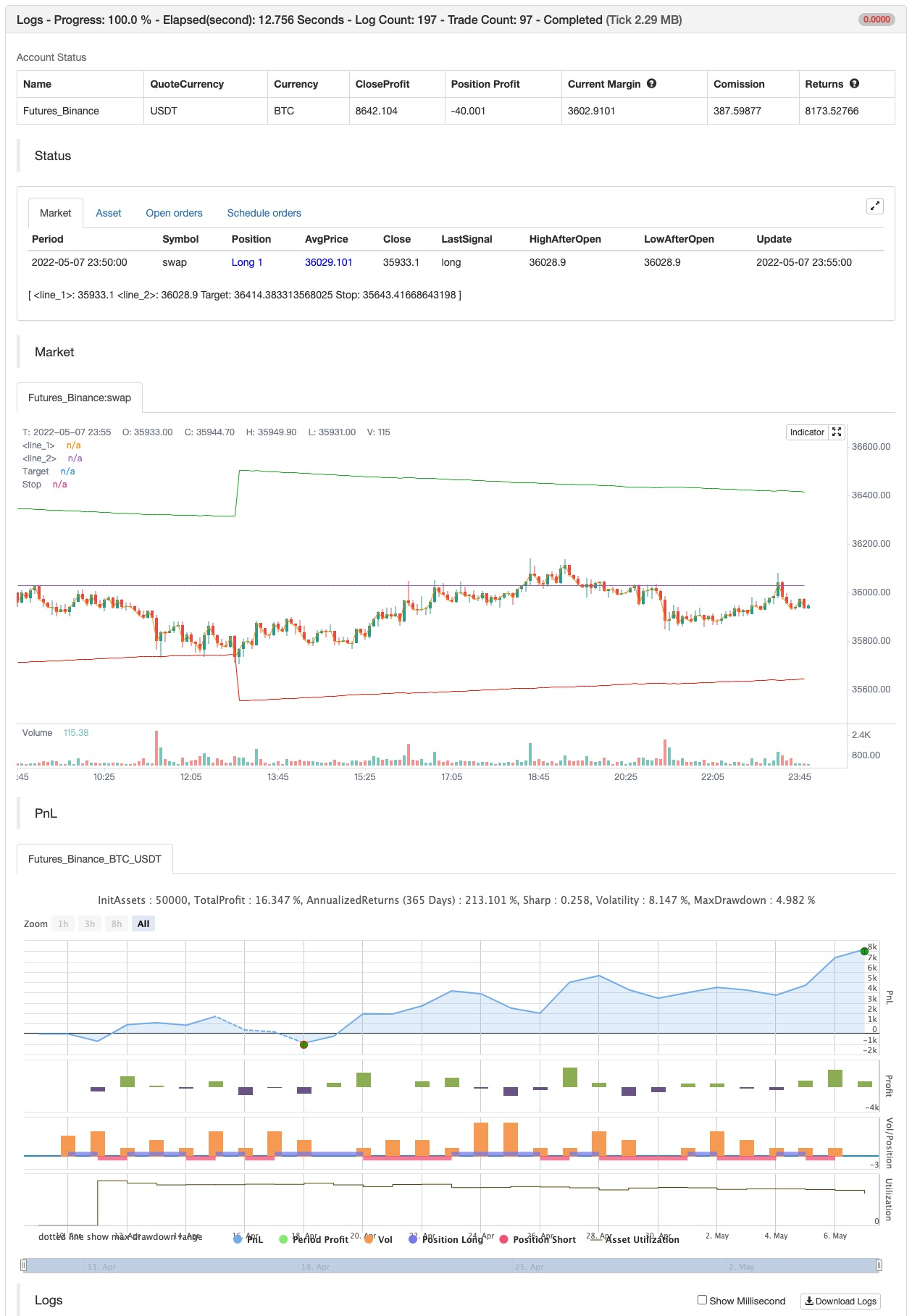# Fibonacci Progression With Breaks

Author: 张超, Date: 2022-05-17 10:24:46
Tags: ATR

This indicator highlights points where price significantly deviates from a central level. This deviation distance is determined by a user-set value or using a multiple of a period 200 Atr and is multiplied by successive values of the Fibonacci sequence.

Settings

Method: Distance method, options include “Manual” or “Atr” Size: Distance in points if the selected method is “Manual” or Atr multiplier if the selected method is “Atr” Sequence Length: Determines the maximum number of significant deviations allowed.

Usage

The indicator allows highlighting potential reversal points, but it can also determine trends using the central level, with an uptrend detected if the central level is higher than its previous value and vice versa for a downtrend.

When an uptrend is detected, and the price deviates significantly upward from it a first checkmark will be highlighted alongside the Fibonacci sequence used as a multiplier, if the price deviates downward, a cross will be shown instead, then the distance threshold will be multiplied by the next value in the Fibonacci sequence.

If the price deviates from the central level such that the length of the sequence is greater than the user set Sequence Length, a break label will be shown alongside a new central level with a value determined by the current closing price, while the Fibonacci multiplier will be reset to 1.

Upper and lower extremities made from the central level and threshold distance are highlighted and can be used as support and resistances.

backtest```/*backtest
start: 2022-04-10 00:00:00
end: 2022-05-07 23:59:00
period: 5m
basePeriod: 1m
exchanges: [{"eid":"Futures_Binance","currency":"BTC_USDT"}]
*/

//@version=5
indicator("Fibonacci Progression With Breaks [LUX]",overlay=1,max_labels_count=500,max_lines_count=500)
method = input.string('Atr',options=['Atr','Manual'],inline='inline1')
size   = input(1.,'',inline='inline1')
max    = input(3,'Sequence Length')
//----
var fib = array.from(1,1)
var dist = 0.,var avg = 0.,var fib_n = 1,var os = 0

src = close
n = bar_index

if barstate.isfirst
for i = 1 to max
array.push(fib,array.get(fib,i-1) + array.get(fib,i))
//----
if method == 'Atr'
dist := ta.atr(200)*size*array.get(fib,fib_n)
else
dist := size*array.get(fib,fib_n)

fib_n := math.abs(src-avg) > dist ? fib_n+1 : fib_n
avg := nz(fib_n > max+1 ? src : avg,src)
fib_n := fib_n > max+1 ? 1 : fib_n

sell = avg < avg
os := buy ? 1 : sell ? 0 : os

tp = avg != avg ? na : os == 1 ? avg + dist : avg - dist
sl = avg != avg ? na : os == 0 ? avg + dist : avg - dist
//----
css = os == 1 ? #0cb51a : #ff1100
plot0 = plot(src,color=na)
plot1 = plot(avg,color=na)
fill(plot0,plot1,color.new(css,80))
//----
plotshape(sell ? high : na,"Sell Label",shape.labeldown,location.absolute,#ff1100,0,text="S",textcolor=color.white,size=size.tiny)

plot(tp,'Target',#0cb51a,1,plot.style_linebr)
plot(sl,'Stop',#ff1100,1,plot.style_linebr)